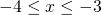# Solution assignment 01 Quadratic inequalities

### Assignment 1

Solve: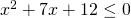### Solution

First we solve the corresponding equation:which can be easily solved by factorizing. We write: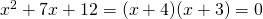and the solutions are: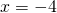or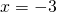The graph of the function in the left-hand side is an 'opens up' parabola, and thus the following values ofsatisfy the inequality: